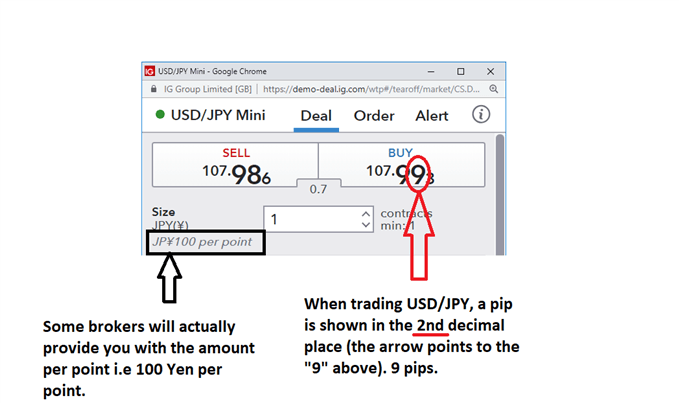## What are pips in forex trading?

“PIP” – which stands for Point in Percentage – is the unit of measure used by forex traders to define the smallest change in value between two currencies. This is represented by a single digit move in the fourth decimal place in a typical forex quote.

For example, if the price of EUR/USD moves from 1.1402 to 1.1403 this would be a one pip or ‘point’ movement.

Example of a pip using the quote to buy EUR/USD

However, not all forex quotes are displayed in this way, with the Japanese Yen being the notable exception. Keep reading to find out more about pips and how they’re used in forex trading, with examples from selected major currency pairs.

## How to calculate the value of a pip?

The pip value is calculated by multiplying one pip (0.0001) by the specific lot/contract size. For standard lots this entails 100,000 units of the base currency and for mini lots, this is 10,000 units. For example, looking at EUR/USD, a one pip movement in a standard contract is equal to \$10 (0.0001 x 100 000).

Being able to calculate the value of a single pip helps forex traders put a monetary value to their take profit targets and stop loss levels. Instead of simply analysing movements in pips, traders can determine how the value of their trading account (equity) will fluctuate as the currency market moves.

It’s important to note that the value of one pip will differ for different currency pairs. This is because the value of one pip will always be shown in the currency of the quote/variable currency and this will differ when trading different currency pairs. When trading EUR/USD, the value of one pip will be displayed in USD, when trading GBP/JPY, this will be in JPY.

## Calculating the value of one pip – EUR/USD pips example

As each currency has its own relative value, it’s necessary to calculate the value of a pip for each particular currency pair.

Keep in mind that forex trading involves set amounts of currency that you can trade. Most brokers offer a standard and a mini contract with the specifications in the table below:

 Type of Contract Contract size (No. of units of the base currency) Standard Lot 100 000 Mini Lot 10 000

The value of one pip for the EUR/USDstandard contract is calculated as follows:

Pip Value = Contract Size x One Pip

Pip Value = 100 000 x 0.0001

Pip Value = \$10

Every one pip move in your favor translates into a \$10 profit and every one pip move that goes against you translates into a \$10 loss. By the same logic, a one pip move in a mini contract translates into a \$1 profit or loss (10,000 x 0.0001).

To help understand pips and pip calculations even further you may want to consider doing some practice calculations on your own.

## Pip Value Conversions

Now, if your account is based in Great British Pounds (GBP), you would have to convert that \$1 (value of a pip for a 10k EUR/USD lot) into Pounds. To do so, just divide the \$1 by the current GBP/USD exchange rate, which at the time of writing is 1.2863. It is necessary to divide here because a Pound is worth more than a US dollar, so I know my answer should be less than 1. 1 divided by 1.2863 is 0.7774 Pounds. So now you know that if you have a Pound based account, and profit or lose one pip on one 10k lot of EUR/USD, you will earn or lose 0.7774 Pounds.

## The exception – USD/JPY pips

When trading major currencies against the Japanese Yen, traders need to know that a pip is no longer the fourth decimal but rather the second decimal. This is because the Japanese Yen has a much lower value than the major currencies.

Looking at the USD/JPY quote below, the ask (buy) price is as much as 107.99 Yen for 1 USD.When trading the mini contracts (10k) and standard contracts (100k) in Japanese Yen, a one pip movement (the value of one pip) will be JPY100 and JPY1000, respectively.